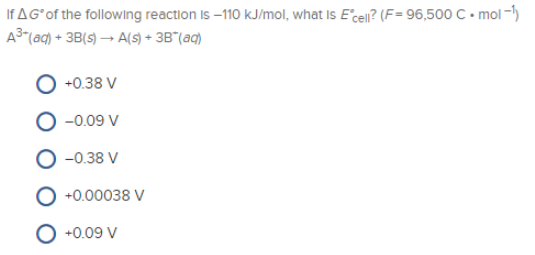# Problem: If ΔG° of the following reaction is -110 kJ/mol. what is E°cell? (F = 96.500 C • mol -1) A3- (aq) + 3B (s) → A (s) + 3B - (aq) (a) + 0.38 V (b) - 0.09 V (c) - 0 38 V (d) + 0.00038 V (e) + 0.09 V

###### FREE Expert Solution
96% (433 ratings)###### Problem Details

If ΔG° of the following reaction is -110 kJ/mol. what is E°cell? (F = 96.500 C • mol -1

A3- (aq) + 3B (s) → A (s) + 3B - (aq)

(a) + 0.38 V

(b) - 0.09 V

(c) - 0 38 V

(d) + 0.00038 V

(e) + 0.09 V